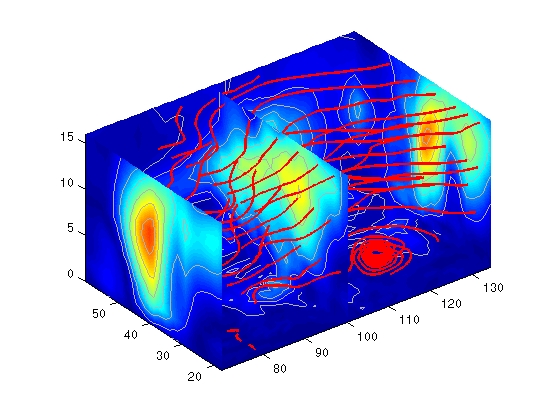3-D VisualizationStream Line Plots of Vector Data

MATLAB includes a vector data set called `wind` that represents air currents over North America. This example uses a combination of techniques:

• Stream lines to trace the wind velocity
• Slice planes to show cross-sectional views of the data
• Contours on the slice planes to improve the visibility of slice-plane coloring

1. Determine the Range of the Coordinates

Load the data and determine minimum and maximum values to locate the slice planes and contour plots (`load`, `min`, `max`).

• ```load wind
xmin = min(x(:));
xmax = max(x(:));
ymax = max(y(:));
zmin = min(z(:));
```

2. Add Slice Planes for Visual Context

Calculate the magnitude of the vector field (which represents wind speed) to generate scalar data for the `slice` command. Create slice planes along the x-axis at `xmin`, `100`, and `xmax`, along the y-axis at `ymax`, and along the z-axis at `zmin`. Specify interpolated face coloring so the slice coloring indicates wind speed, and do not draw edges (`sqrt`, `slice`, `FaceColor`, `EdgeColor`).

• ```wind_speed = sqrt(u.^2 + v.^2 + w.^2);
hsurfaces = slice(x,y,z,wind_speed,[xmin,100,xmax],ymax,zmin);
set(hsurfaces,'FaceColor','interp','EdgeColor','none')
```

3. Add Contour Lines to the Slice Planes

Draw light gray contour lines on the slice planes to help quantify the color mapping (`contourslice`, `EdgeColor`, `LineWidth`).

• ```hcont = ...
contourslice(x,y,z,wind_speed,[xmin,100,xmax],ymax,zmin);
set(hcont,'EdgeColor',[.7,.7,.7],'LineWidth',.5)
```

4. Define the Starting Points for the Stream Lines

In this example, all stream lines start at an x-axis value of 80 and span the range 20 to 50 in the y direction and 0 to 15 in the z direction. Save the handles of the stream lines and set the line width and color (`meshgrid`, `streamline`, `LineWidth`, `Color`).

• ```[sx,sy,sz] = meshgrid(80,20:10:50,0:5:15);
hlines = streamline(x,y,z,u,v,w,sx,sy,sz);
set(hlines,'LineWidth',2,'Color','r')
```

5. Define the View

Set up the view, expanding the z-axis to make it easier to read the graph (`view`, `daspect`, `axis`).

• ```view(3)
daspect([2,2,1])
axis tight```

See `coneplot` for an example of the same data plotted with cones.Accessing Subregions of Volume Data Displaying Curl with Stream Ribbons© 1994-2005 The MathWorks, Inc.